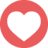# 救我

2016年12月5日 23:082

1. 左邊電壓V，上面X，下面Y，右邊接地=0，I從左邊流入 對地做KCL，(-I)+(X-0)/R+(Y-0)/2R=0 => Y=2IR-2X 對V做KCL，I+(X-V)/2R+(Y-V)/R=0 => 3V-2IR=X+2Y=4IR-3X => X=2IR-V 對X做KCL，(V-X)/2R+(Y-X)/3R+(0-X)/R=0 => 3V=11X-2Y=15X-4IR=26IR-15V => 9V=13IR => V/I=(13/9)R 2. 先把電容C做laplace變成阻抗Z=1/sC，之後跟上面一樣的算法。 不過注意算完要弄成1/(sXC)，X就是等效的容值倍數。 -麥

KCL說穿了就是流進的電流等於流出的電流啦XDDD 就是對各個節點作電路分析而已 算容值偷吃步一下XDDD 換成阻抗真的差很多www -麥

△→Y參考這個B21 喔好吧 我蠢只會這個(๑•̀ㅂ•́)و✧

effect和equal是一樣的意思XDD 然後我發現我前面算錯了QQ -麥